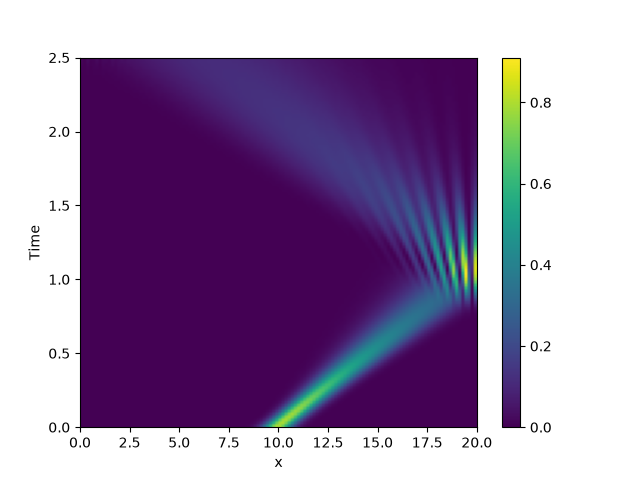# 2.18. Schrödinger’s Equation

This example implements a complex PDE using the PDE. We here chose the Schrödinger equation without a spatial potential in non-dimensional form:

$i \partial_t \psi = -\nabla^2 \psi$

Note that the example imposes Neumann conditions at the wall, so the wave packet is expected to reflect off the wall.from math import sqrt

from pde import PDE, CartesianGrid, MemoryStorage, ScalarField, plot_kymograph

grid = CartesianGrid([[0, 20]], 128, periodic=False)  # generate grid

# create a (normalized) wave packet with a certain form as an initial condition
initial_state = ScalarField.from_expression(grid, "exp(I * 5 * x) * exp(-(x - 10)**2)")
initial_state /= sqrt(initial_state.to_scalar("norm_squared").integral.real)

eq = PDE({"ψ": f"I * laplace(ψ)"})  # define the pde

# solve the pde and store intermediate data
storage = MemoryStorage()
eq.solve(initial_state, t_range=2.5, dt=1e-5, tracker=[storage.tracker(0.02)])

# visualize the results as a space-time plot
plot_kymograph(storage, scalar="norm_squared")


Total running time of the script: (0 minutes 6.966 seconds)